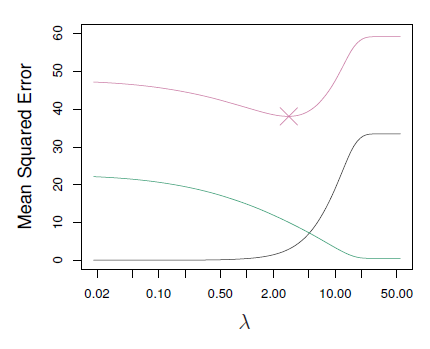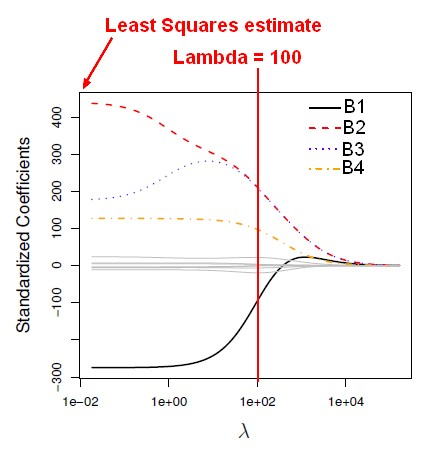# Statistical Learning - Lasso

Lasso is a shrinkage method.

Ridge regression doesn't actually select variables by settings the parameters to zero. Lasso is a more recent technique for shrinking coefficients in regression that overcomes this problem.

In the case of the lasso, the $l_1$ penalty has the effect of forcing some of the coefficient estimates to be exactly equal to zero when the tuning_parameter $\lambda$ is sufficiently large (whereas it's not the case with ridge regression)

Hence, much like best subset selection, the lasso performs variable selection. It's a combination of both:

Lasso comes from a paper that Robert Tibshirani wrote in 1996.

## Shrinkage Penalty

The least squares fitting procedure estimates the regression parameters using the values that minimize RSS. In contrast, the lasso (as ridge regression) estimates the regression parameters (lasso coeffecients $\hat{\beta}^L_{\lambda}$ minimizing the RSS with a penalty term.

$$\begin{array}{cll} \href{RSS}{RSS} & + & \underbrace{\lambda\sum^p_{j=1}|\beta_j|}_\text{penalty term}} \\ \underbrace{\sum^n_{i=1}(y_i-\beta_0- \sum^p_{j=1}{\beta_j x_{ij}})^2}_RSS} & + & \underbrace{\lambda\sum^p_{j=1}|\beta_j|}_\text{penalty term}} \end{array}$$

This computation is a convex optimization.

The algorithm will try to make the RSS small but at the same time, the penalty term is going to push it in the other direction penalizing coefficients which get too large. The more non-zero a coefficient is, the larger the penalty term is. The larger the coefficients are, the bigger the penalty price is.

It's basically fit versus the size of the coefficients.

That penalty is called a shrinkage penalty because it's going to encourage the parameters to be shrunk toward 0.

## Penalty Term

Whereas on ridge regression, the penalty is the sum of the squares of the coefficients, for the Lasso, it's the sum of the absolute values of the coefficients. It's a shrinkage towards zero using an absolute value rather than a sum of squares.

And this is called an L1 penalty. By analogy to the L2 penalty, the L1 penalty is just the some sum of the absolute values. It's a norm, but it's called the L1 norm, rather than the L2 norm.

In statistical parlance, the lasso uses an $l_1$ (pronounced “ell 1”) penalty instead of an $l_2$ penalty. The $l_1$ norm of a coefficient vector $\beta$ is given by

$$||\beta||_1 = \sum |\beta_j|$$

## Tuning parameter $lambda$

As in ridge regression, selecting a good value for the Tuning parameter $\lambda$ for the lasso is critical; cross-validation is again the method of choice.

At the point where the black coefficient becomes 0, you can throw away all variables , and just retain the three remaining, the blue, red, and orange.

The right graph shows the shrinkage but in the other direction.

## Sparsity

Lasso will set coefficients to 0 exactly if that feature's not important and lambda's large enough. There's a term for this. It's called sparsity.

So the Lasso used what's called sparse models. Models which only involve a subset of the variables.

The lasso yields sparse models that is, models that involve only a subset of the variables.

Why by using an absolute value for the penalty, we get a sparsity property. Ridge regression and the lasso will minimize the RSS with the penalty term as constraint. which means that the shrinkage problem will find the smallest RSS within a budget defined by:

• a circle for ridge aggression (square)
• a diamond for lasso (absolute value). The absolute value's going to be a constraint region that has sharp corners.

The solution will be the first place the RSS contours hit the constraint region.

In high dimensions, with the lasso, you have edges and corners that makes the dimanond. And along an edge or a corner, if you hit there, you get a 0. So this is, geometrically, why you get sparsity in the Lasso.

## P and n

With the progress in convex optimization and fast computation, the Lasso problem can be solved for very large values of p and n.

With variables that might be in the tens of thousands, you can solve it on a standard desktop computer in less than a minute.

## R

Glm

Recommended PagesData Mining - (Function|Model)

The model is the function, equation, algorithm that predicts an outcome value from one of several predictors. During the training process, the models are build. A model uses a logic and one of several...Data Mining - Elastic Net Model

In statistics and, in particular, in the fitting of linear or logistic regression models, the elastic net is a regularized regression method that linearly combines the L1 and L2 penalties of the lasso...Statistics - (Shrinkage|Regularization) of Regression Coefficients

Shrinkage methods are more modern techniques in which we don't actually select variables explicitly but rather we fit a model containingall p predictors using a technique that constrains or regularizes...Statistics - Ridge regression

Ridge regression is a shrinkage method. It was invented in the '70s. The least squares fitting procedure estimates the regression parameters using the values that minimize RSS. In contrast, the...